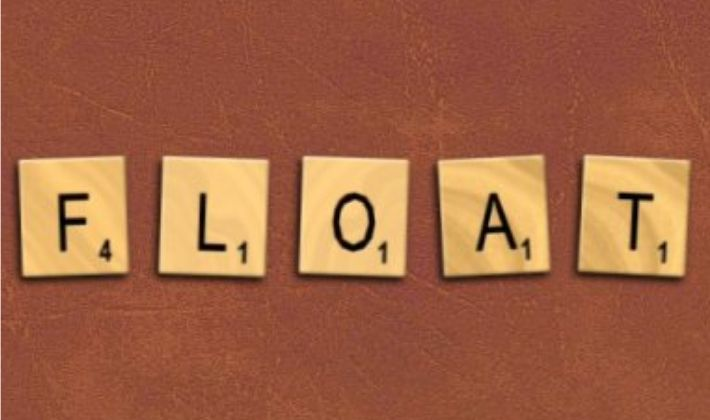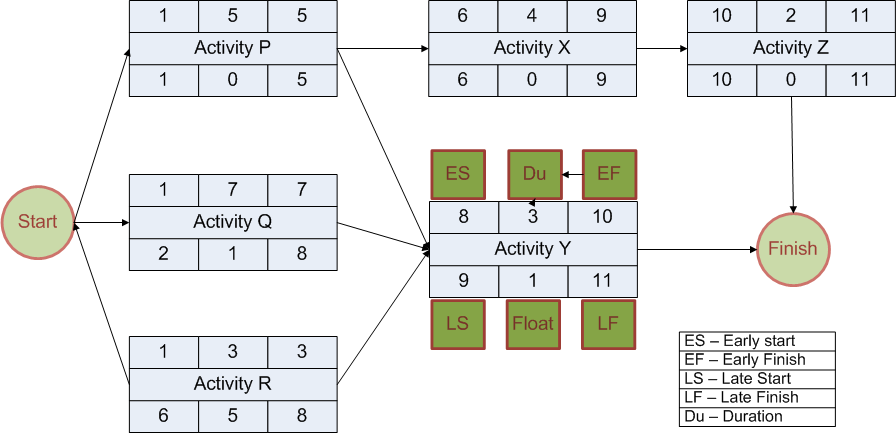Total Float is the maximum amount of time an activity can be delayed without delaying the project whereas Free Float is the amount of time an activity can be delayed without impacting the Early Start date of any of its immediate successors.

Total Float and Free Float are used in Critical Path Method (CPM) to determine schedule flexibility of an activity. They are independent of each other, which means that an activity can have different amount of free and total float.

I have written this article to explain the difference between Total Float and Free Float in project Management. It provides a complete and in-depth explanation of different types of floats using examples, calculations and diagrams. It also includes definitions and formulas that can be used for solving PMP certification exam mathematical questions.

Note: I have written this post assuming that you have the basic understanding of project schedule network diagrams and Critical Path Method (CPM) concepts. You should read about Schedule Network Diagrams and Critical Path Analysis before continuing further.

## Total Float

Total Float is the maximum amount of time an Activity can be delayed without delaying the Project.

e.g. if Total Float (TF) for an Activity Alpha is ‘n’ days, it means Activity Alpha can be delayed by ‘n’ days without impacting the finish date of the project. If Alpha is delayed by ‘n’ days of less then its successor(s) might get delayed but the finish date would not be impacted.

You can refer to Max Wideman’s Glossary for a few other standard definitions.

### Total Float Formula

Total Float can be calculated by using either of the following formulas:

TF = LF – EF

OR

TF = LS – ES

Both the formulas will produce same result.

Let us understand the concept with the help of a small example. Refer to the following network diagram. It is solved using 1 method of CPM.As per the above diagram:

1. There are 6 activities viz. P, Q, R, X, Y, and Z.
2. P, Q, and R start as soon as the project starts.
3. X is successor of P.
4. Y is successor of P, Q and R.
5. Z is successor of X.

In the above diagram TF for Activity P and Activity Q are 0 and 1 respectively.

## Free Float

Free Float is the the amount of time an Activity can be delayed without impacting the Early Start date of any of its Immediate Successors.

e.g. Consider Activity Alpha & Activity Beta that have a Finish to Start dependency between them i.e. Activity Beta is successor of Activity Alpha. If Activity Alpha has a Free Float (FF) of ‘n’ days then it means that Activity Alpha can be delayed by a maximum of ‘n’ days without impacting the Early Start of Activity Beta.

You can refer to Max Wideman’s Glossary for a few other standard definitions.

### Free Float Formula

Free Float can be calculated by using one of the following formulas:

FF = min(ES of Successors) – (ES of Activity in Question) – (Duration of Activity in Question)

OR

FF = min(ES of Successors) – (EF of Activity in Question)

The first formula is a generic formula and will work for both 0 and 1 method in critical path analysis but the second one will work only if you have used 1 method.

Refer to the above network diagram again.

FF for Activity X is 0 as any delay in X will delay ES of Z.

FF Activity Q is 0 as any delay in Q will delay ES of Y. Notice that TF of Activity Q is 1.

FF Activity R is 4. The ES of Y will change only if R is delayed by more than 4 days. Notice that TF of Activity Q is 5.

## Total Float vs Free Float

Total Float and Free Float are very different concepts. Although they are calculated for each activity, they serve entirely different purpose. Total Float is concerned with the project finish date whereas Free Float is concerned with the immediate predecessor(s) of an activity.

## Difference Between Total Float and Free Float

Here are a few pertinent points about TF:

1. Buffer (Contingency Reserve) and TF are not same. Buffer is purposefully introduced in an activity whereas TF is due to its placement in the Network Diagram.
2. TF is calculated separately for each activity. TF is mentioned in lower middle box of each activity in the above diagram.
3. The term TF is a misnomer as it says ‘Total’ but it is calculated for each activity and not for the entire path.
4. TF is shared among the activities that are on the same path in a Network Diagram. If one activity uses TF, the TF of other activities will proportionately reduce. e.g. If Q uses 1 unit of TF on Path QY, then Y will have no TF.
5. The activities on the Critical Path have least amount of TF. Generally, it is zero but it could be negative also. In the above diagram, PXZ is the Critical Path.

Here are a few pertinent points about FF:

1. Lag and FF are not same. Lag is purposefully introduced in an activity whereas FF is due to its placement in the Network Diagram.
2. FF is calculated separately for each activity. FF is generally not mentioned in the Network Diagram.
3. FF is useful when there is an imposed start date for a Successor activity.
4. All the successor activities should be considered while calculating the FF.
5. TF and FF of an Activity can be different.
6. The above formula for FF is applicable for Finish to Start relationships only.

### Final Words

Float is also referred to as Slack. In some Project Management literature, you may find terms like Total Slack and Free Slack. Some books also use Project Float of just Float in place of Total Float although the using the former term is incorrect.

Both the floats are very important for determining the final project schedule. They are used to determine the amount of flexibility in the project schedule. They can also be used for analyzing the constraint dates and determining the schedule risks.

### PMP Exam Formulas

I have also compiled a PMP Formulas Cheat Sheet. It contains 45 formulas and 57 abbrviations. It will help you in your exam prep. It is the best and most comprehensive cheat sheet based on the PMBOK Guide 6th edition. You can download it free of cost for your studies.

If you are looking beyond a cheat sheet, then I would suggest you to buy detailed PMP Exam Formula Study Guide by Cornelius Fichtner. It contains detailed explanations of all the formulas along with examples and 105 practice questions.

Disclosure: This article contains affiliate links - it means that, if you buy from any of these links, then I will receive a small commission that would help me in maintaining this blog for free. However, for you, there is no extra cost. I recommend only those products that I believe will definitely help the certification aspirants.

## Similar Posts

1. Looks like your description over activity D in the middle, careful!, It’s the activty Duration and it’s not Early Start or ES. Am I right?

Robert A. Romero

2. Looks like your description over activity D in the middle, careful!, It’s the activty Duration and it’s not Early Start or ES. Am I right?

Robert A. Romero

August 13, 2011 8:50 PM

1. Praveen Malik says: